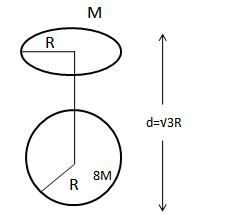Courses

# MCQ: Gravitation, Class 11 NEET Notes | EduRev

## NEET : MCQ: Gravitation, Class 11 NEET Notes | EduRev

The document MCQ: Gravitation, Class 11 NEET Notes | EduRev is a part of NEET category.
All you need of NEET at this link: NEET

Ques: A uniform ring of mass M & radius R is placed directly above a uniform sphere of mass 8M & radius R. The centre of ring is at a distance of d=√3R from the centre of the sphere. The gravitational attraction between the sphere and ring is

Solution:Gravitational field due to ring at a distance

d=3–√Rd=3R on its axis is

E=GMd/(R^2+d^2)^3/2

=√3GM/8R^2

Force on the sphere = mass of sphere × E

=8ME

Hence, Force =√3GM^2/R^2

Offer running on EduRev: Apply code STAYHOME200 to get INR 200 off on our premium plan EduRev Infinity!

,

,

,

,

,

,

,

,

,

,

,

,

,

,

,

,

,

,

,

,

,

,

,

,

;